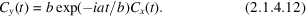International
Tables for
Crystallography
Volume B
Reciprocal space
Edited by U. Shmueli

International Tables for Crystallography (2006). Vol. B. ch. 2.1, pp. 192-193   | 1 | 2 |

## Section 2.1.4.1. Characteristic functions

U. Shmuelia* and A. J. C. Wilsonb

aSchool of Chemistry, Tel Aviv University, Tel Aviv 69 978, Israel, and bSt John's College, Cambridge, England
Correspondence e-mail:  ushmueli@post.tau.ac.il

#### 2.1.4.1. Characteristic functions

| top | pdf |

The average value of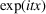is very important in probability theory; it is called the characteristic function of the distributionand is denoted by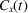or, when no confusion can arise, by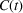. It exists for all legitimate distributions, whether discrete or continuous. In the continuous case it is given by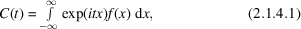and is thus the Fourier transform of. In many cases it can be obtained from known integrals. For example, for the Cauchy distribution,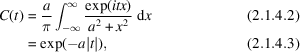and for the normal distribution,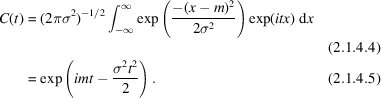Since the characteristic function is the Fourier transform of the distribution function, the converse is true, and if the characteristic function is known the probability distribution function can be obtained by the use of Fourier inversion theorem,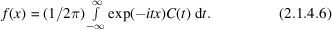An alternative approach to the derivation of the distribution from a known characteristic function will be discussed below.

The most important property of characteristic functions in crystallography is the following: if x and y are independent random variables with characteristic functionsand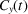, the characteristic function of their sum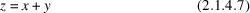is the product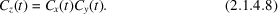Obviously this can be extended to any number of independent random variables.

When the moments exist, the characteristic function can be expanded in a power series in which the kth term is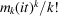. If the power series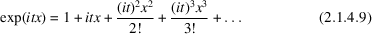is substituted in equation (2.1.4.1), one obtains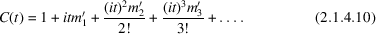The moments are written with primes in order to indicate that equation (2.1.4.10)is valid for moments about an arbitrary origin as well as for moments about the mean. If the random variable is transformed by a change of origin and scale, say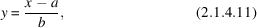the characteristic function for y becomes